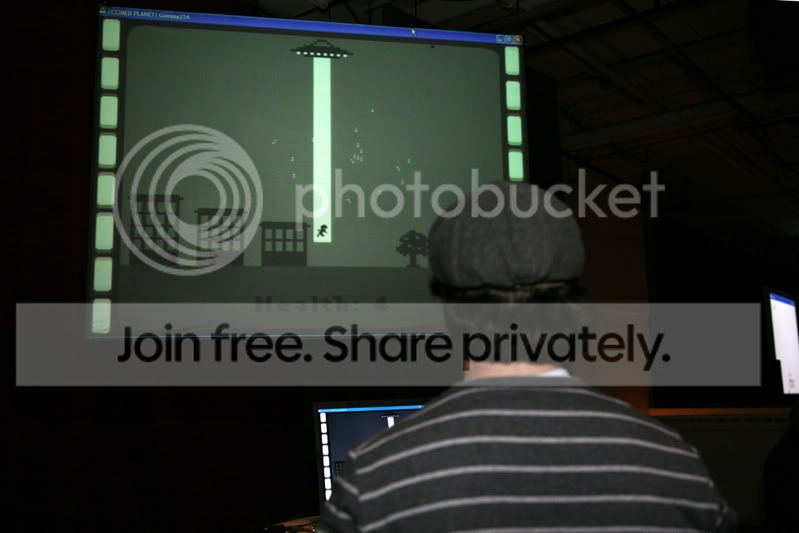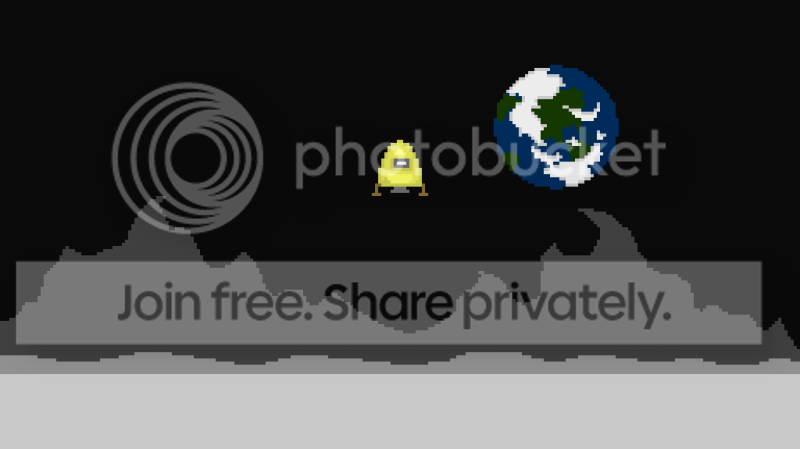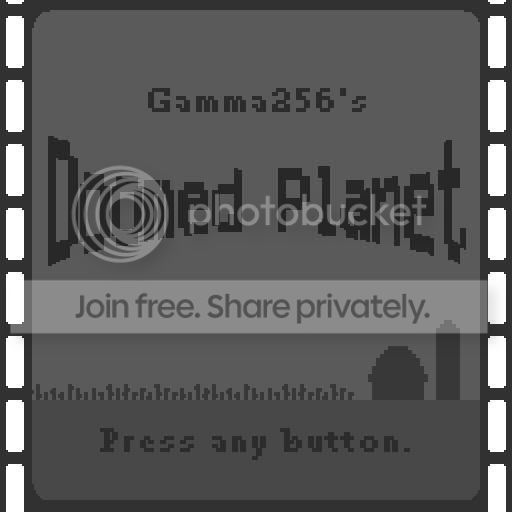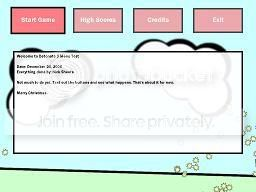-=+=- -=+=- -=+=- -=+=- -=+=- -=+=- -=+=- -=+=- -=+=- -=+=- -=+=- -=+=- -=+=- -=+=- -=+=- -=+=- -=+=- -=+=- -=+=- -=+=- -=+=- -=+=- -=+=- -=+=- -=+=- -=+=- -=+=- -=+=- -=+=- -=+=- (c) WidthPadding Industries 1987 0|507|0 -=+=- -=+=- -=+=- -=+=- -=+=- -=+=- -=+=- -=+=- -=+=- -=+=- -=+=- -=+=- -=+=- -=+=- -=+=- -=+=- -=+=- -=+=- -=+=- -=+=- -=+=- -=+=- -=+=- -=+=- -=+=- -=+=- -=+=- -=+=- -=+=- -=+=-

### blanko1324

View Profile, Send Private Message
Recent Posts, Showcases, Articles, Code Snippets, Links, Wed.Workshop, Blog

### Latest Blog Entries

blanko1324# My Gamma4 Entry

31st January 2010

Video Included...

blanko1324# Small Space Project

6th September 2009

This is my latest little side project:

After taking the Summer to watch the first season of the original Star Trek, I was inspired to make a game, not where you fly a space ship, but where you actually command it. This is my "Small Space Project&qu<snip>

blanko1324# Swaying Trees

7th July 2008

Still messing around with my "Nice Little Map System", I decided to replace the marker trees with something a little less...static.

[thumb]https://socoder.net/uploads/15/PAXtreeSS.PNG[/t<snip>

blanko1324# Re-Work

12th June 2008

Back to the Puzzle Game...

blanko1324# Nice Little Map System

26th March 2008

What I'm working on...

blanko1324# Looked Like a Fun Party...

2nd December 2007Aw well, there's always next year.

Cool stuff, eh? See the rest of the pictures here.

### Latest Showcases

blanko1324# To The Moon!

18th February 2010A Game for Gamma 4

blanko1324# Spaceship Sim

14th January 2010A small space project I've been fooling around with. (Inspired by Star Trek)

blanko1324# Shambo

16th June 2008A Game of Super Strategy Maybe. (VER 0.7)

blanko1324# Doomed Planet!

28th October 2007...has NOT been rejected!

blanko1324# Detonate 3

24th December 2006Updated 1/21/07